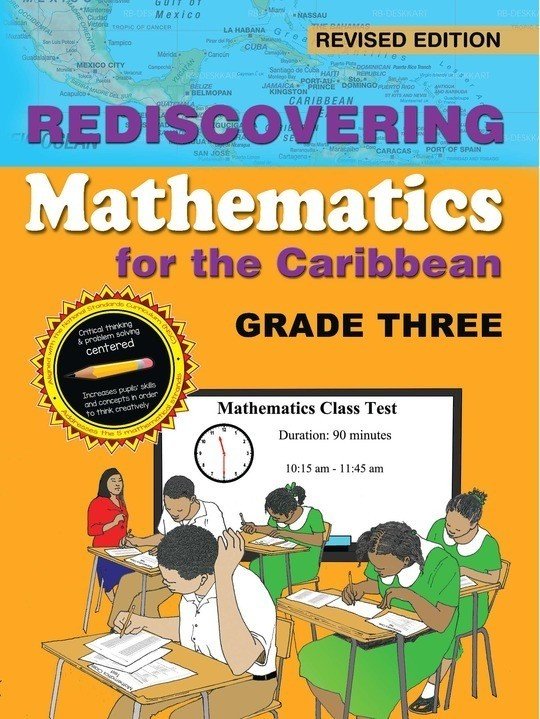\$8.99

# Rediscovering Mathematics for the Caribbean: Grade 3

US\$ 8.99
The publisher has enabled DRM protection, which means that you need to use the BookFusion iOS, Android or Web app to read this eBook. This eBook cannot be used outside of the BookFusion platform.
Book Description

Rediscovering Mathematics for the Caribbean: Grade Three, is the fifth of a comprehensive series of eight textbooks which covers the requirements of the Mathematics syllabuses for Primary Schools in the Caribbean. The text provides a firm foundation in Mathematics for primary school pupils utilizing a learner-centred approach.

Some Key Features:

Basic numeracy skills are emphasized and topics are carefully introduced.
Numerals, exercises and illustrations provide pupils with a lot of practice, thereby enhancing their understanding of mathematical concepts.
Concepts are expressed in simple, clear and easy to understand language that is appropriate to the grade level and subject.
Meaningful connections are made to real life situations.
Other features include activities that provide opportunities for students to engage in critical thinking and problem solving, and students are required to perform tasks that extend and reinforce knowledge and skills learnt. The language used throughout the series is simple and straightforward and pupils and teachers should find the learning and teaching experience fun and quite enjoyable.

• Cover Page
• Title Page
• Acknowledgement
• Preface
• Dedication
• Contents
• NUMBER
• Place Value
• Reviewing 3-Digit Numbers
• Hundreds, Tens and Ones
• Using an Abacus to Write 3-Digit Numbers
• Thousands
• Adding Using the Number Line
• Adding Numbers Horizontally and Vertically Without and With Regrouping
• Comparing Numbers
• Comparing Bumbers Within 1,000
• Expanded Notation with Renaming
• Subtraction
• Subtraction Using the Number Line
• Subtraction of Numbers Within 10,000
• Subtraction With Regrouping (Renaming) in Hundreds and Thousands
• Subtraction With Regrouping (Renaming) When Necessary - ones, Tens, Hundreds and Thousands
• Regrouping in Ones, Tens, Hundreds and Thousands
• Cardinal Numbers
• Ordinal Numbers
• Odd or Even?
• Let's Review
• The Calculator
• Using a Calculator
• The Dozen (12)
• Greater Than or Less Than, Equal To
• Rounding Off Numbers
• Estimation and Approximation
• The Roman Numeral System
• Money
• What is Money?
• Change to Cents Only
• Subtraction of Money
• Subtraction of Money with Regrouping (Renaming)
• Multiplication with Renaming
• Division of Money
• Money- Writing Bills
• Let's Learn to Skip - Count
• Multiplication
• Doing Multiplication by Using Arrays
• Multiplication Using Pairs
• Multiplying by Using a Table or a Chart
• Commutative Multiplication
• Multiplying 3-Digit Numbers by 1-Digit Number Without Renaming
• Multiplying 3-Digit Numbers by 1-Digit Number with Renaming
• Zero Property of Multiplication
• Multiplying on a Number Line
• More Practice
• Division
• Division-The Opposite of Multiplication
• Divided by 0 and Divided by 1
• Long Division
• Division with Regrouping in Hundreds, Tens and Ones
• Division With Remainder
• Division: Two-Step Word Problems
• Complete Sequences
• Creating Patterns
• Using Numbers & Extending Number Patterns
• Pattern in Geometry
• Growing and Shrinking Patterns
• Number Pattern on the Hundred Chart
• What is a Fraction?
• Identifying Fractions
• Fractions: Halves, Thirds and Quarters
• Equivalent Fractions
• Fractions - Part of a Set
• Finding the Fraction of a Set
• Comparing and Ordering Fractions
• Comparing and Ordering Fractions with same Denominators
• Comparing and Ordering Fractions with different Denominators
• More on Equivalent Fractions
• Proper Fractions
• Improper Fractions
• Mixed Numbers
• Addition and Subtraction of Fractions with Like Denominators
• Addition and Subtraction of Fractions with Unlike Denominators
• Finding the Unknown
• Finding 'n' in a Mathematical Sequence
• Bar Modelling
• MEASUREMENT
• The Metric System
• Length - Instruments Used for Measuring
• Metre
• Subtraction of Length
• Multiplication of Length
• Division of Length
• Mass - Gram
• What's my Mass?
• Addition and Subtraction of Mass
• Multiplication of Mass
• Division of Mass
• Problem Solving
• Estimate and Verify Mass/Weight of Various Objects
• Measuring Kilograms and Grams
• Capacity/Volume
• Litres
• Multiplication of Litres
• Problem Solving
• Division of Litres
• More Practice
• Telling Time
• Minutes Past the Hour
• Minutes to the Hour
• What Time is it?
• Subtraction: When the Greater Minutes are at the Bottom
• Conversion of Hours to Minutes
• Days and Weeks
• Addition of Days and Weeks
• Subtraction of Days and Weeks
• Multiplication of Days and Weeks
• Division of Days and Weeks
• Temperature
• GEOMETRY
• Polygons
• Regular or Irregular Polygons
• Perimeter
• Area
• Calculating the Area of Shapes
• Area of a Rectangle
• Area of a Square
• Lines, Paths, Shapes
• Lines and Curves
• Angles
• Naming Angles
• Closed and Open Figures
• Curves or Paths
• Solid and Plane Shapes
• Cone & Pyramids
• Line of Symmetry
• HANDLING DATA AND PROBABILITY
• Statistics - Handling Data
• Pictograph
• Bar Graph
• Pie Chart
• Probability
• ASSESSMENT
• EXTENDED THINKING
• GLOSSARY
• INDEX
• DO YOU KNOW YOUR MULTIPLICATION TABLES?
• GOOD TO KNOW
The book hasn't received reviews yet.
You May Also Like